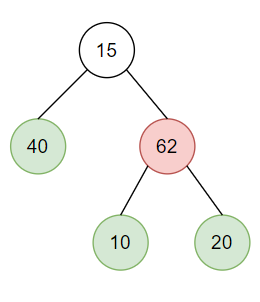New update is available. Click here to update.

# Closest Leaf To Given Node In Binary Tree

Last Updated: 23 Dec, 2020
Difficulty: Moderate

## PROBLEM STATEMENT

#### Ninja is presently at the node ‘X’. The only exit points of the maze are the leaf nodes of the tree. You need to tell him the distance to the nearest exit point from his current location. This will help him decide which path he should take in order to escape from the maze.

##### Example:``````Suppose, Ninja is stuck at node 62. The possible exit points in the maze are: 40, 10, and 20. From all the possible exit points the closest ones are 10 and 20 which are at a distance of 1 unit.
``````
##### Input format:
``````The very first line of input contains an integer 'T' denoting the number of test cases.

The first of every test case contains the value of node ‘X’, i.e. Ninja’s current position.

The second line of every test case contains elements of the binary tree in the level order form. The line consists of values of nodes separated by a single space. In case a node is null, we take -1 on its place.
``````
##### Example:
``````The level order input for the tree depicted in the above image would be :

15 40 62 -1 -1 10 20 -1 -1 -1 -1
``````

#### Explanation :

``````Level 1 :
The root node of the tree is 15.

Level 2 :
Left child of 15 = 40
Right child of 15 = 62

Level 3 :
Left child of 40 = null (-1)
Right child of 40 = null (-1)
Left child of 62 = 10
Right child of 62 = 20

Level 4 :
Left child of 10 = null (-1)
Right child of 10 = null (-1)
Left child of 20 = null (-1)
Right child of 20 = null (-1)

15
40 62
-1 -1 10 20
-1 -1 -1 -1
``````
##### Output format:
``````For each test case, return the distance to the nearest exit point from Ninja’s current location.
``````
##### Note :
``````1. Node ‘X’ will always be a unique value and will always be present in the tree.

2. You don't need to print anything, it has already been taken care of. Just implement the given function.
``````
##### Constraints:
``````1 <= T <= 100
1 <= N <= 3000
1 <= DATA <= 10^5, DATA !=-1

Where 'N' denotes the number of nodes in the tree and 'DATA' denotes the node values of the tree.

Time limit: 1 sec
``````## Approach 1

The important point to understand here is that the closest leaf can either be a descendent of the given node ‘X’ or can be reached through one of the ancestors of the given node ‘X’. The idea is to traverse the given tree in preorder until the given node ‘X’ is found. While traversing the tree to find the node ‘X’, store the ancestors of node ‘X’ in an array. These ancestor nodes act as a root for subtrees, which we’ll traverse later to find if there’s any other leaf node closer to node ‘X’ (other than the leaf nodes present in the subtree rooted at ‘X’).

When the given node ‘X’ is found in the tree. Calculate the distance of the closest leaf node in the subtree rooted at ‘X’. Store the result obtained in the previous step. Now, traverse through the previously-stored ancestor nodes array. For every subtree rooted with an ancestor, find the distance of the closest leaf node to the given node ‘X’. The minimum of these distances gives us the final answer.